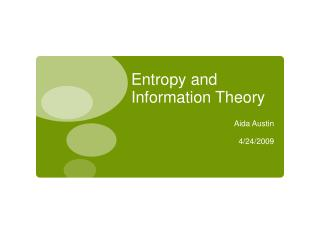# Entropy and Information Theory - PowerPoint PPT PresentationDownload PresentationEntropy and Information Theory

Entropy and Information TheoryDownload Presentation## Entropy and Information Theory

- - - - - - - - - - - - - - - - - - - - - - - - - - - E N D - - - - - - - - - - - - - - - - - - - - - - - - - - -
##### Presentation Transcript

1. Entropy and Information Theory Aida Austin 4/24/2009

2. Overview • What is information theory? • Random variables and entropy • Entropy in information theory • Applications • Compression • Data Transmission

3. Information Theory • Developed in 1948 by Claude • E. Shannon at Bell Laboratories • Introduced in “A Mathematical • Theory of Communication'' • Goal: Efficient transmission of • information over a noisy • network • Defines fundamental limits on • compression needed for reliable • data communication Claude E. Shannon

4. Random Variables • A random variable is a function. • Assigns numerical values to all possible • outcomes (events) • Example: A fair coin is tossed. Let X • represent a random variable. • Possible outcomes:

5. Entropy in Information Theory • Entropy is the measure of the average information • content missing from a set of data when the value • of the random variable is not known. • Helps determine the average number of bits • needed for storage or communication of a signal. • As the number of possible outcomes for a random • variable increases, entropy increases. • As entropy increases, information decreases • Example: MP3 sampled at 128 kbps has higher • entropy rate than 320 kbps MP3

6. Applications • Data Compression • MP3 (lossy) • JPEG (lossy) • ZIP (lossless) • Cryptography • Encryption • Decryption • Signal Transmission Across a Network • Email • Text Message • Cell phone

7. Data Compression • “Shrinks” the size of a signal/file/etc. to • reduce cost of storage and transmission • Smaller data size reduces the possible • outcomes of the associated random • variables, thus decreasing the entropy of • the data. • Entropy - minimum number of bits needed • to encode with a lossless compression. • Lossless (no data lost) if the rate of • compression = entropy rate

8. Signal/Data Transmission • Channel coding reduces bit error and bit loss • due to noise in a network. • As entropy increases, the likelihood of valuable • information transmitted decreases. • Example: Consider a signal composed of • random variables. • We may know the probability of certain values • being transmitted, but we do not know the exact • values will be received unless the transmission • rate = entropy rate.

9. Questions?

10. Resources http://www.gap-system.org/~history/PictDisplay/Shannon.html http://en.wikipedia.org/wiki/Information_entropy http://en.wikipedia.org/wiki/Bit_rate http://lcni.uoregon.edu/~mark/Stat_mech/thermodynamic_entropy_and_information.html http://www.data-compression.com/theory.html